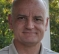## Bit width in a digital delta sigmaStarted by 6 years ago5 replieslatest reply 6 years ago248 views

Hi,

In the attached DSM MOD-2_DSM, how does one determine the bit-widths? Is there analytical relation of bitwidth in relation to the input bitwidth? How does one go about optimizing the DSM in terms of power and bitwidth? Any reference dealing with such questions would be helpful.

Thanks

[ - ]Hello Deba,

In any data converter, i.e. ADC or DAC, the number of bits, ENOB, or dynamic range would be a function of required SNR, sampling frequency, adjacent channel interferer, and sampling frequency/clock.

Data converters' textbook would discuss the relationship between SNR and number of bits.  That is the first part of what you need.

The second part, you need to understand the system requirements that you are working on.

Then you can analyze and draw conclusion for bit-width.

Best regards,

Shahram

https://ortenga.com/

[ - ]Hi Shahram

The Digital DSM is part of a two point injection modulator. The topology of the DSM was finalized from system level simulations in Matlab. But it is an idealized Z-domain model meaning infinite range for state variables. I am facing difficulty in translating that to the fixed point modulator which I have shown in the attached figure.

Thanks

[ - ]Hi Deba,

Looking at the block diagram that you have shared with us, I conclude that all the bit precision at each stage is set up to preserve the required number of bits so that no information is lost, except for the quantizer which is the only place we would expect to see the precision lost.

The inputs are 16 bit signed I would expect.  The first addition would give 17 bits under normal circumstances, but the subsequent subtraction involves the 18 bits of reconstruction after quantization.  The subtraction maintains the 18 bits, as we would expect the result of the subtraction to be equal to or smaller than its two inputs.

The 18 bit register is then added to a 17 bit register output that would not normally result in 19 bits, but it then uses 19 bits for the following 19 bit number subtraction.  It appears that there will only ever be 17 meaningful bits remaining, so I conclude that no bits are lost in preserving only 17 bits.

After the 17 bit register, there is a 2^13 added for rounding before the quantizer (discard 14 bits) from 18 to 4 bits.  These 4 bits are then shifted back up to form the 18 bit feedback for the second order sections.

The lower path is simply 2 delays in registers that preserve the original input samples, but maintain the time alignment with the output of the DSM.

The only question that I can see related to preserving precision is in the e_sub_Q quantization error, where I'm not sure that I see why it should not be 18 bits.

I have no idea if this is useful, but it is my assessment.

So the answer to your questions is that - in fixed point arithmetic - all the stages have exactly the number of bits to preserve the full resolution of their expected values respectively, except for the quantization stage, which will lose precision virtually by the definition of DSM.

David

[ - ]Hi David,

Your bitwidth analysis is correct if there was no feedback but I see accumulators in the design so bitwidth calculation may not be that straight forward

[ - ]